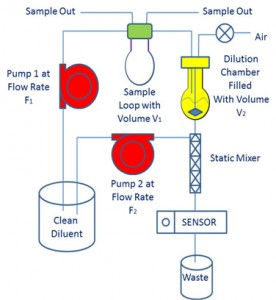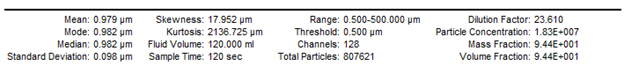• Cart
• LanguageChoose Language
• CurrencyChoose CurrencyTo deal with extremely concentrated colloidal suspensions, Particle Sizing Systems developed a patented two-stage Autodilution scheme. Besides being able to perform dilutions up to 200,000x within minutes, the two-stage dilution scheme allows for automatic sampling through the use of a sample-loop capture system. This extends the capabilities of the Single Particle Optical Sizing (SPOS) technique to online analysis and the addition of an autosampler. Certain industrial colloidal systems like paints and inks are extremely concentrated and require a lot of dilution in order to employ SPOS. The figure displays a schematic of the Two-Stage Autodilution scheme as it would be used in the AccuSizer 780 APS :

The two-stage Autodilution scheme uses a two position fill-inject valve which contains a loop of known volume, V1. In the fill position, sample is allowed to flow through the loop and out the valve thereby filling the loop with concentrated sample. The sample could be under pressure from a reactor or process stream or a pump could pull sample through. After the loop is full, the valve is put into the inject position allowing pump 1 to flow diluent into the loop at flow rate F1, carrying the sample into a dilution chamber of known volume, V2. It is important to note that pump 1 can generate variable flow rates. This accomplishes the first stage of dilution. The dilution factor of this stage is V2/V1. Typical values for V1and V2are 0.5 ml and 30 ml, leading to a first stage dilution factor of 60X.

Simultaneous to this, pump 2 starts to flow clean diluent into the static mixer at flow rate F2. F2is much larger than F1. Even after the sample is injected into the dilution chamber, pump 1 continues to operate, flowing in clean diluent into the dilution chamber. Since the dilution chamber is closed, liquid exits into the static mixer at the same flow rate as pump 1. There it mixes with the flow from pump 2. This represents the second stage of dilution. The dilution factor can be expressed as:

2ndstage dilution factor = 1+ F2/F1

As can be seen from the above equation, the second stage dilution can be controlled by changing the flow rate of pump 1. In this two-stage scheme, instead of diluting continuously like in the exponential Autodilution arrangement, the flow rate of pump 1 can be increased or decreased to obtain a steady-state count rate, as monitored by the light obscuration sensor and set by the user. That is why this fluidics arrangement is ideal for online analysis. As might be expected by a process stream, the concentration of particles will change with time. The two-stage dilution scheme can adjust the overall dilution factor as required from measurement to measurement as the sample concentration changes. This two-stage mixed-flow method of dilution not only provides a large range of dilution factors that can be adjusted based on the concentration of sample, but once a set point concentration is achieved, sample can be delivered to the SPOS sensor at a nearly constant concentration for relatively long periods of time. This permits a large number of sample particles to be counted, resulting in enhanced statistical accuracy of the resulting particle size distribution.

A count rate profile for a two-stage Autodilution measurement is shown:

For this measurement, the set point concentration was 7,000 particles/ml. The first F1the fluidics settled on produced a particle concentration of 17,000 particles/ml. The search algorithm lowered the F1flow rate, thus increasing the 2ndstage dilution factor, which produced a particle concentration of 7,060 particles/ml, within range of the set point. This was the F1used for the actual data collection. As can be seen, the particle concentration remained constant throughout the entire measurement.Just like in the Exponential Autodilution scheme, the concept of Dilution Factor can be utilized. As before, Dilution Factor or DF is defined at the ratio of particles introduced into the system over the number of particles counted in the measurement. Phrasing it in terms of the two-stage dilution scheme, DF would be the number of particles in the loop over the number of particles counted in a measurement. Since the volume of the loop and the number of particles counted are known and the DF is measured, the particle concentration can be expressed as:

Co= (Particles Counted x DF)/V1

Keeping in mind the expressions for the first stage dilution factor and the second stage dilution factor, the following equation for DF can be developed:

DF = V2/(F1x T)

Where T is the data collection time

This is a simplified expression as it assumes that the particle concentration in the dilution chamber will not change with time. However, this is not always true. As pump 1 is continuously flowing clean diluent into the dilution chamber, the particle concentration in the dilution chamber falls exponentially (see Autodilution ). In the case of the extremely concentrated colloidal dispersions that the two-stage Autodilution system would be employed on, this exponential decrease is extremely slow and the above expression for DF holds. In the case where the concentration will fall off significantly during the data collection time T, an exponential correction factor needs to be added into the expression for DF.

An example of a two-stage Autodilution measurement can be seen below:The particle size distribution is from a commercial dispersion of 1 micron polystyrene latex spheres. These particles are very narrowly dispersed. They were introduced into the system as a 1:1000 predilution which is easy to make on the laboratory bench. The measured particle concentration was 1.83 x 107particles/ml well within the expected particle concentration of 1.9 +/- 0.2 x 107particles/ml. The fluidics were setup with a 1 ml sample loop volume (V1= 1 ml). The software chose a flow rate for pump 1 of 0.65 ml/min (F1= 0.65 ml/min). With an F2= 60 ml/min, the second stage dilution factor will be 93.4. The number of particles counted in a 120 second collection time was 807,621 which is considerably more than could be counted with the Exponential Autodilution. Furthermore, the known solids loading of this dispersion is 1%. Since all the particles in the dispersion are countable, the solids volume recovery should be 100%. The 780 recovered 94.4% of the solids volume. Both the particle concentration and % volume recovery numbers confirm the accuracy the Two-Stage Autodilution approach. Overall the Two-Stage Autodilution diluted the sample by a factor of 2,600.

The actual results from the printout can be seen here.

In conclusion, the Two-Stage Autodilution is shown to be accurate. It works best with extremely concentrated dispersions. The system is able to monitor and change dilution factors so has to handle samples that change with time, for example, sample undergoing homogenization. It provides a quick and accurate way to obtain large number of particle counts so as to generate statistically accurate tail information.